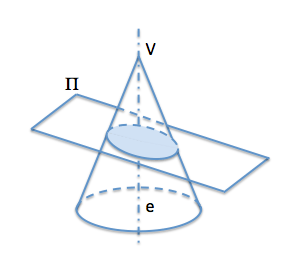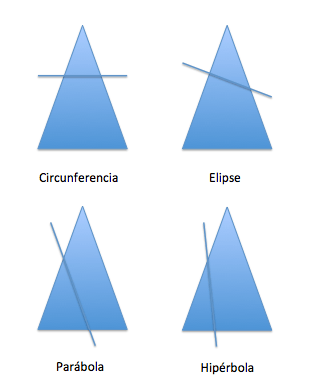Metric geometry: Curves : ConicalAmong the most important curves are studied in geometry is called “Conic curves”.

These curves can be analyzed under different conceptual approaches, from the point of view of their spatial conception, metrics, projective, analytics …. come to be known as the “Definition of conic curves”.

Another common name for these curves is the “Conic Sections” because the first definition given for them, by Apollonius of Perge, was from the sections in a cone of revolution. This first definition, based on a spatial model, known as “first definition of conic”.

Se denomina conic section (o simplemente conic) every curve intersection of a cone and a plane.We can see this same figure in a representation in which the producing section plane is perpendicular to the drawing plane. In this representation we see that there are two angles that characterize the cone and the direction of the axis of the plane “and” thereof:

• Alfa: half top angle “V..” the cono.Determina the angle between the generatrix of the cone with the axis “and”
• Beta: angle of the plane with the axis “and” ConeDepending on the position of the plane of the conical surface, this will cut their generating, atodas least one all the least of Ella, determining their own curves all points, with a point at infinity or unfit two points (at infinity) respectively.

Depending on the alpha and beta angles we find the following cases:

• alfa < beta If the half-angle at the apex is smaller than the angle of the plane with the axis, the curve is a ellipse. As a particular case if the plane is perpendicular to the axis curve is a circumference.
• alfa = beta If the cone angles are equal is generated parable
• alfa > beta If is greater than the half angle formed between the plane and the axis, the curve is a hipérbola.The conic sections are important in astronomy: two massive bodies interacting according to the law of universal gravitation, their trajectories describe conic sections if their center of mass at rest is considered. If you are relatively close ellipses described, if you stray too far described hyperbolas or parabolas.(W)

We will see in detail each of these curves to submit new definitions based on metric properties or projective.

Metric geometry